﻿ 通用有限元模拟抗滑桩或桩板墙计算

# 通用有限元模拟抗滑桩或桩板墙计算General Finite Element Calculation of Anti-Slide Pile or Pile Wall

Abstract: With the development of economy and the increasing demand of landscape and modeling for structural engineering, there are many restrictions on the calculation of special-shaped structure through conventional software, which is mainly limited by the software modules or models, so it is impossible to calculate the variable cross-section or special-shaped structure. In this paper, through research and simulation, the general finite element simulation of rock horizontal con-straint and beam element simulation of anti slide pile (or pile sheet wall) are introduced, in this paper are verified by comparison between the simulation calculation of spa2000 and midas Civil software and Li-zheng geotechnical software. Verify the correctness and reliability of the stiffness calculation method of rock soil joints proposed in this paper.

1. 引言

2. 通用有限元模型建模技巧

1) 滑坡推力分布模拟：桩上滑坡推力均布荷载q的计算

$q=P×L/h$ (式-1)

L——抗滑桩中心间距(m)；

h——抗滑桩的悬臂端长度(m)。

2) 岩质地基弹簧刚度系数模拟

${K}_{si}=K\ast {B}_{P}/n$，其中 $i=2,\cdots ,i-1$ (式-2)

${K}_{si}=K\ast {B}_{P}/\left(2n\right)$，其中 $i=1,i$ (式-3)

K——岩体抗力系数(kN/m3)；

n——每延米节点分段数；

BP——桩的计算宽度(m)。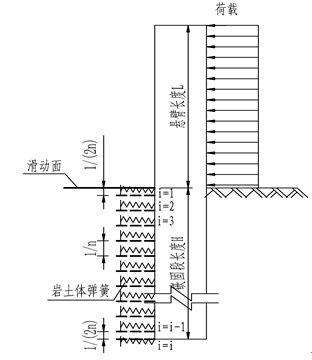Figure 1. Conversion calculation model of spring stiffness coefficient of foundation

《铁路路基支挡结构设计规范》(TB10025-2019)第13.1.2条  桩的截面尺寸应根据滑坡推力的大小、桩间距以及锚固段地基的横向容许承载力等因素确定。抗滑桩的截面形状宜为矩形。桩最小边宽度不宜小于1.25 m。

3. 项目概况

4. 各软件计算结果对比分析

4.1. 理正抗滑桩计算结果

4.2. SAP2000计算结果

4.3. midas计算结果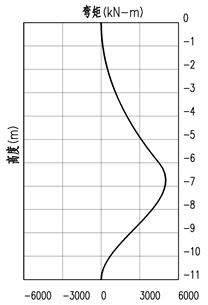Figure 2. Bending moment diagram (kN∙m)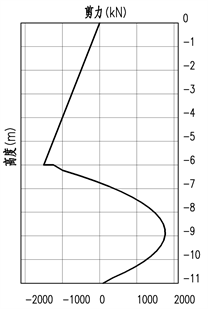Figure 3. Shear diagram (kN)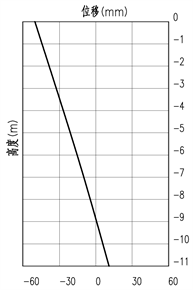Figure 4. Displacement diagram (mm)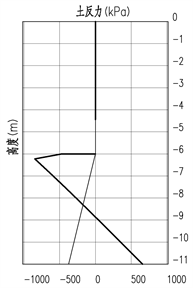Figure 5. Soil inverse force diagram(kN)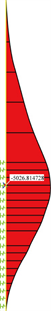Figure 6. Bending moment diagram (kN∙m)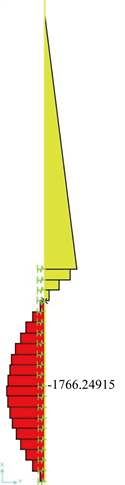Figure 7. Shear diagram (kN)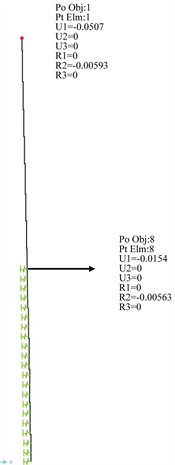Figure 8. Displacement diagram (mm)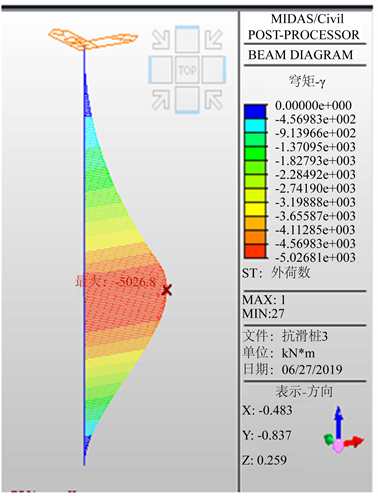Figure 9. Bending moment diagram (kN∙m)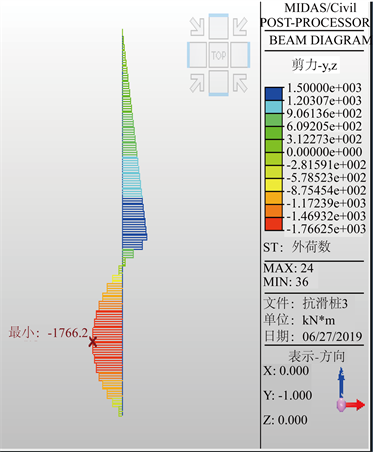Figure 10. Shear diagram (kN)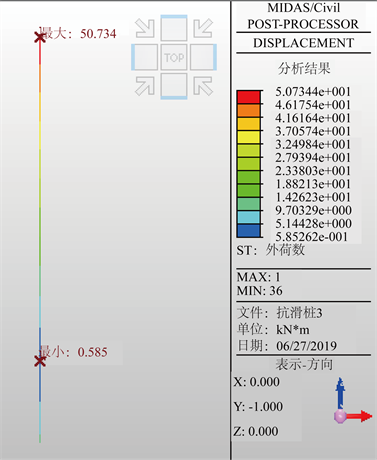Figure 11. Displacement diagram (mm)

4.4. 各软件计算结果对比(表1)Table 1. Comparison of calculation results

5. 结论

 中华人民共和国行业标准. TB 10025-2019铁路路基支挡结构设计规范[S]. 北京: 中国铁道出版社有限公司, 2019.

 中华人民共和国建筑行业标准. JCJ 94-2008建筑桩基技术规范[S]. 北京: 中国建筑工业出版社, 2008.

 中华人民共和国交通行业标准. JTG D30-2015公路路基设计规范[S]. 北京: 人民交通出版社股份有限公司, 2015.

Top